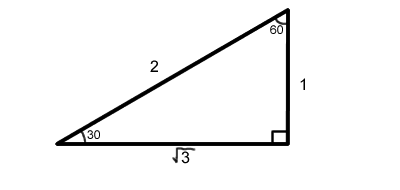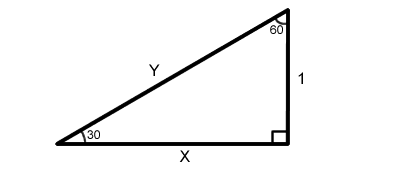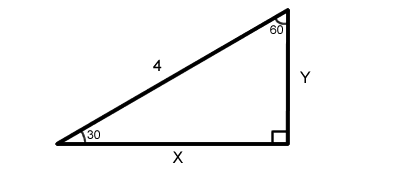# Properties of a 30-60-90 Right Triangle

### A special kind of triangle

A 30-60-90 right triangle (literally pronounced "thirty sixty ninety") is a special type of right triangle where the three angles measure 30 degrees, 60 degrees, and 90 degrees. The triangle is significant because the sides exist in an easy-to-remember ratio: 1:$$\sqrt{3}$$:2. That is to say, the hypotenuse is twice as long as the shorter leg, and the longer leg is the square root of 3 times the shorter leg. You might also remember it as "X, 2X, and X roots of 3", which is how I remember it, but then you have to remember that 2X is actually the longest side, not X roots of 3.

Which side is which? The side opposite the 30 degree angle will have the shortest length. The side opposite the 60 degree angle will be $$\sqrt{3}$$ times as long, and the side opposite the 90 degree angle will be twice as long. The triangle below diagrams this relationship. Remember that the longest side will be opposite the largest angle, and the shortest opposite the smallest angle.We can use the relationship between the angles and the sides of a 30-60-90 triangle to find missing angles or side lengths. Take a look at this example:

### Example 1

Given the 30-60-90 triangle below, find the lengths of the missing sides:Since this is a 30-60-90 right triangle, we know that the sides exist in the proportion 1:$$\sqrt{3}$$:2. The shortest side, 1, is opposite the 30 degree angle. Since side X is opposite the 60 degree angle, we know that it is equal to $$1*\sqrt{3}$$, or about 1.73. Finally, side Y is opposite the right angle, and it is twice the shortest side, or 2.

### Where does the formula come from?

Is this just another made-up math formula? No! This is just an application of basic trigonometry. For the example above, we could have taken the sine of the left-most angle: sin(30) = 1/2. Because sine gives us the ratio of opposite over hypotenuse, we would know that the hypotenuse would have to be 2. Basically, the whole reason a 30-60-90 triangle is easy to solve is because the sine and cosine of those angles are also very simple.

### Example 2

Use the same principles to solve for the unknown variables X and Y.The known side is 4, and that is the longest side. Remember how the longest side is twice the shortest side for a 30-60-90 triangle? That means Y must be 2!

Now, we can find the remaining side. Since the side opposite the 60 degree angle equals the shorter side times the square root of 3, we can calculate X equals $$2*\sqrt{3}$$.

### Summary

For a right triangle with angles measuring 30, 60 and 90 degrees, the sides will have lengths in a ratio of 1:$$\sqrt{3}$$:2, as shown in this diagram:### More Help

As always, you can ask your specific questions in our math help message board, search Google, or check out this lesson on 30-60-90 triangles, or this one. Or, to calculate the sides and angles of a triangle, use the interactive tool below: